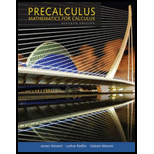Chapter 2.2, Problem 33E### Precalculus: Mathematics for Calcu...

7th Edition
James Stewart + 2 others
ISBN: 9781305071759

#### Solutions

Chapter
Section### Precalculus: Mathematics for Calcu...

7th Edition
James Stewart + 2 others
ISBN: 9781305071759
Textbook Problem

# Graphing Piecewise Defined Functions Sketch a graph of the piecewise defined function.33. f ( x ) = { 0 if    x < 2 1 if   x ≥ 2

To determine

To sketch: The graph of given piecewise defined function.

Explanation

The given function is,

f(x)={0ifx<21ifx2

In the given inequality function two functions generate with condition,

f(x)=0(forx<2) ,

f(x)=1(forx2)

### Still sussing out bartleby?

Check out a sample textbook solution.

See a sample solution

#### The Solution to Your Study Problems

Bartleby provides explanations to thousands of textbook problems written by our experts, many with advanced degrees!

Get Started

## Additional Math Solutions

#### Find more solutions based on key concepts